# Properties of Solutions Solutions Solutions are homogeneous mixtures

• Slides: 41Properties of SolutionsSolutions • Solutions are homogeneous mixtures of two or more pure substances. • In a solution, the solute is dispersed uniformly throughout the solvent.Solutions The intermolecular forces between solute and solvent particles must be strong enough to compete with those between solute particles and those between solvent particles.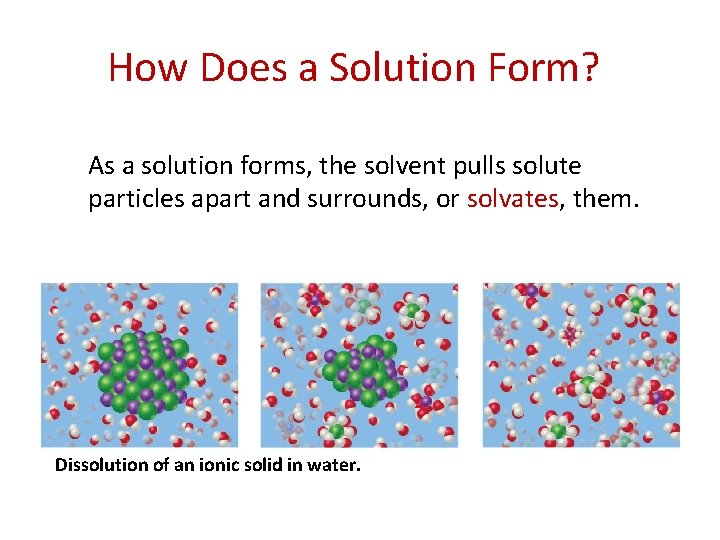How Does a Solution Form? As a solution forms, the solvent pulls solute particles apart and surrounds, or solvates, them. Dissolution of an ionic solid in water.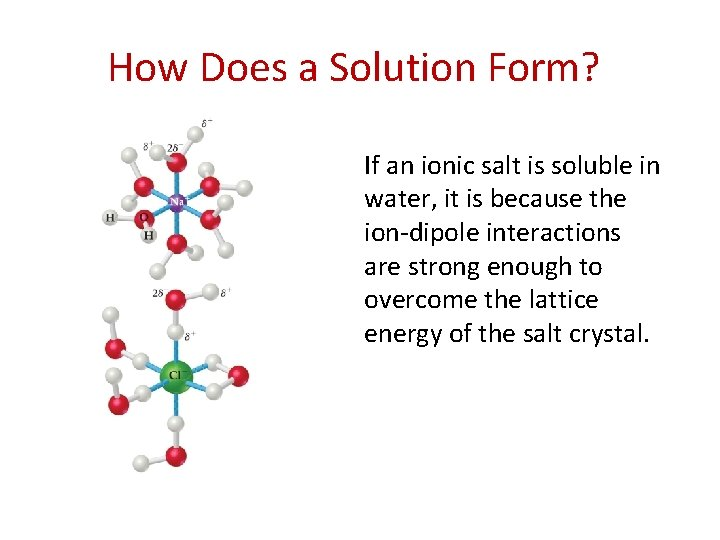How Does a Solution Form? If an ionic salt is soluble in water, it is because the ion-dipole interactions are strong enough to overcome the lattice energy of the salt crystal.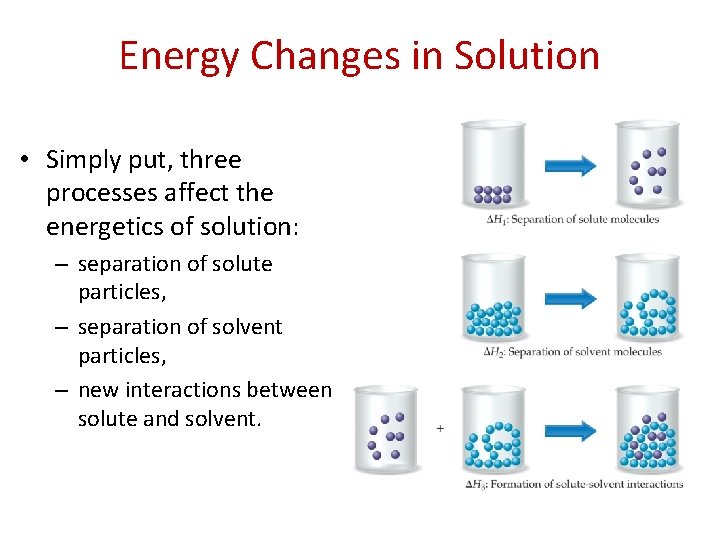Energy Changes in Solution • Simply put, three processes affect the energetics of solution: – separation of solute particles, – separation of solvent particles, – new interactions between solute and solvent.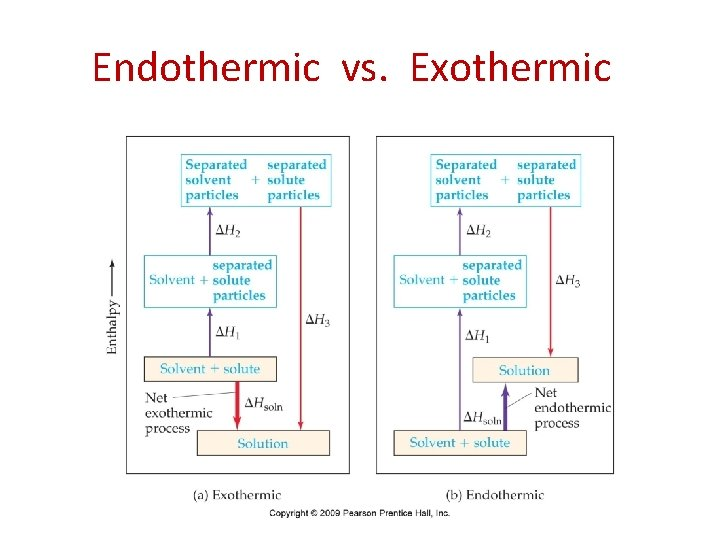Endothermic vs. ExothermicInstant Ice Pack Ammonium nitrate instant ice pack are often used to treat athletic injuries.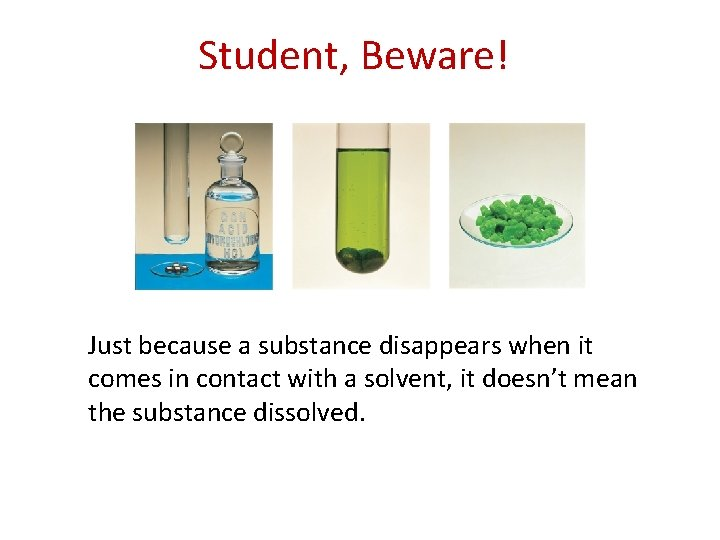Student, Beware! Just because a substance disappears when it comes in contact with a solvent, it doesn’t mean the substance dissolved.Student, Beware! • Dissolution is a physical change — you can get back the original solute by evaporating the solvent. • If you can’t, the substance didn’t dissolve, it reacted.Factors Affecting Solubility • Chemists use the axiom “like dissolves like. " – Polar substances tend to dissolve in polar solvents. – Nonpolar substances tend to dissolve in nonpolar solvents. Nonpolar liquids tend to be insoluble in polar liquids.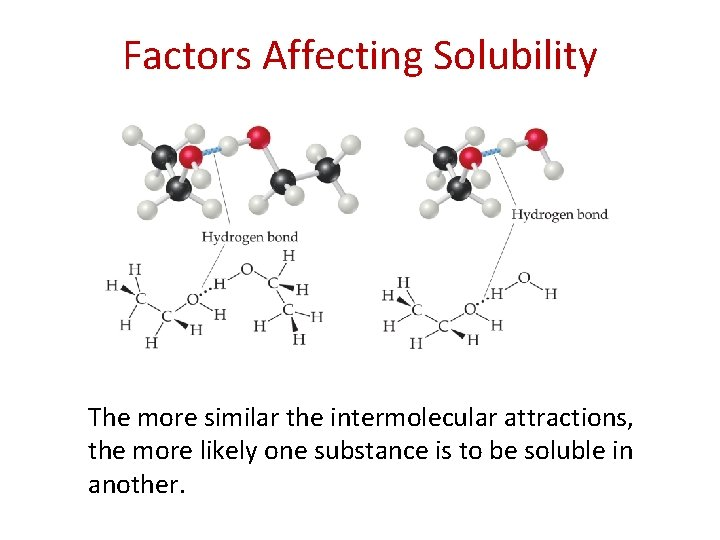Factors Affecting Solubility The more similar the intermolecular attractions, the more likely one substance is to be soluble in another.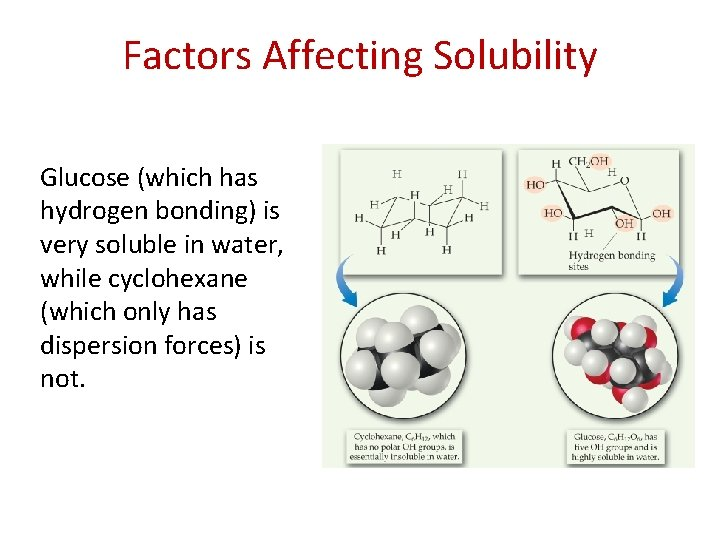Factors Affecting Solubility Glucose (which has hydrogen bonding) is very soluble in water, while cyclohexane (which only has dispersion forces) is not.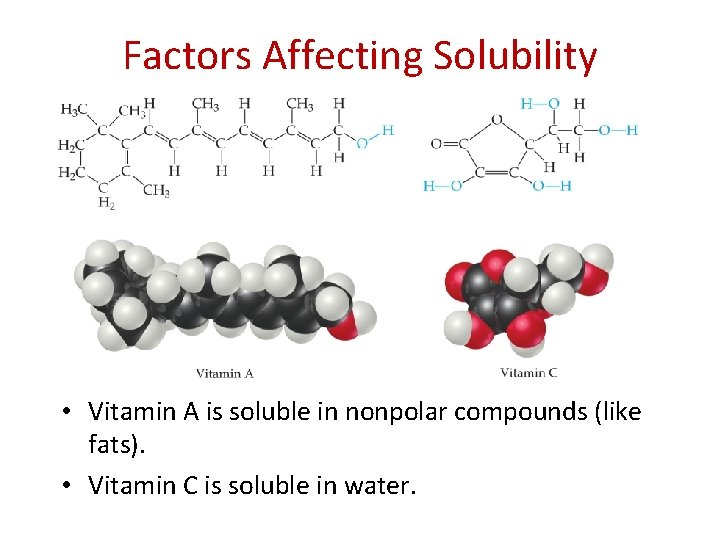Factors Affecting Solubility • Vitamin A is soluble in nonpolar compounds (like fats). • Vitamin C is soluble in water.Gases in Solution • The solubility of liquids and solids does not change appreciably with pressure. • The solubility of a gas in a liquid is directly proportional to its pressure.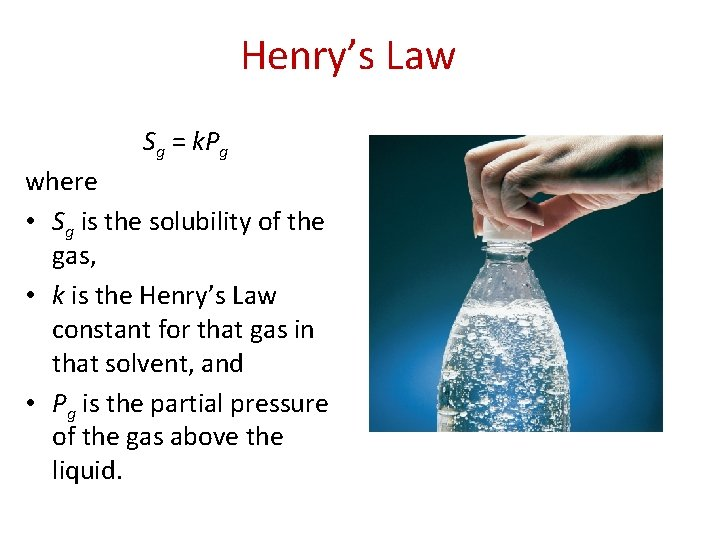Henry’s Law Sg = k. Pg where • Sg is the solubility of the gas, • k is the Henry’s Law constant for that gas in that solvent, and • Pg is the partial pressure of the gas above the liquid.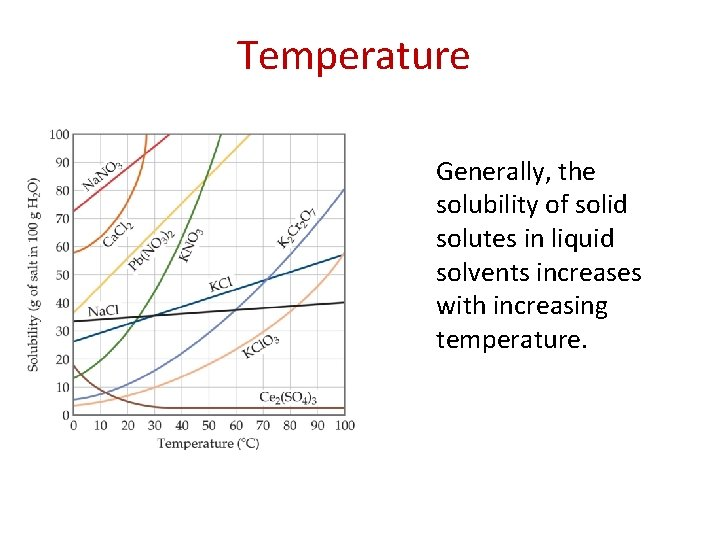Temperature Generally, the solubility of solid solutes in liquid solvents increases with increasing temperature.Temperature • The opposite is true of gases. – Carbonated soft drinks are more “bubbly” if stored in the refrigerator. – Warm lakes have less O 2 dissolved in them than cool lakes.Colligative Properties • Changes in colligative properties depend only on the number of solute particles present, not on the identity of the solute particles. • Among colligative properties are – Vapor pressure lowering – Boiling point elevation – Melting point depression – Osmotic pressure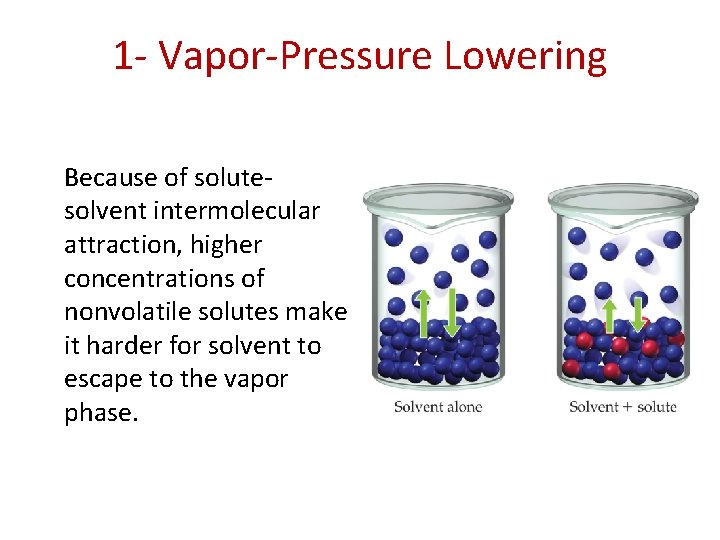1 - Vapor-Pressure Lowering Because of solutesolvent intermolecular attraction, higher concentrations of nonvolatile solutes make it harder for solvent to escape to the vapor phase.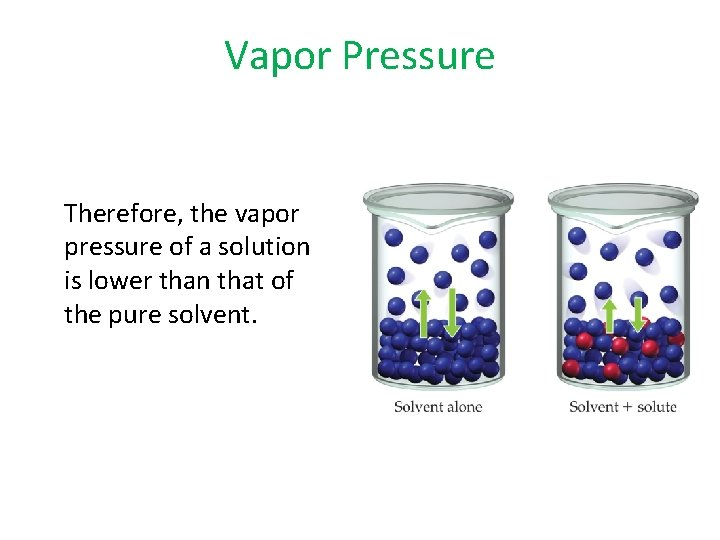Vapor Pressure Therefore, the vapor pressure of a solution is lower than that of the pure solvent.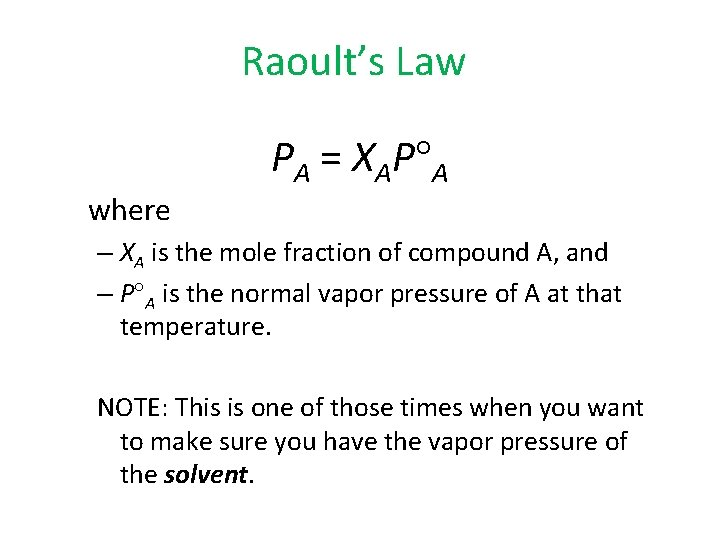Raoult’s Law PA = XAP A where – XA is the mole fraction of compound A, and – P A is the normal vapor pressure of A at that temperature. NOTE: This is one of those times when you want to make sure you have the vapor pressure of the solvent.Glycerin (C 3 H 8 O 3) is a nonvolatile nonelectrolyte with a density of 1. 26 g/m. L at 25 °C. Calculate the vapor pressure at 25 °C of a solution made by adding 50. 0 m. L of glycerin to 500. 0 m. L of water. The vapor pressure of pure water at 25 °C is 23. 8 torr, and its density is 1. 00 g/m. L. The vapor pressure of the solution has been lowered by 0. 6 torr relative to that of pure water.2 - Boiling Point Elevation • The change in boiling point is proportional to the molality of the solution: Tb = Kb m where Kb is the molal boiling point elevation constant, a property of the solvent. Tb is added to the normal boiling point of the solvent.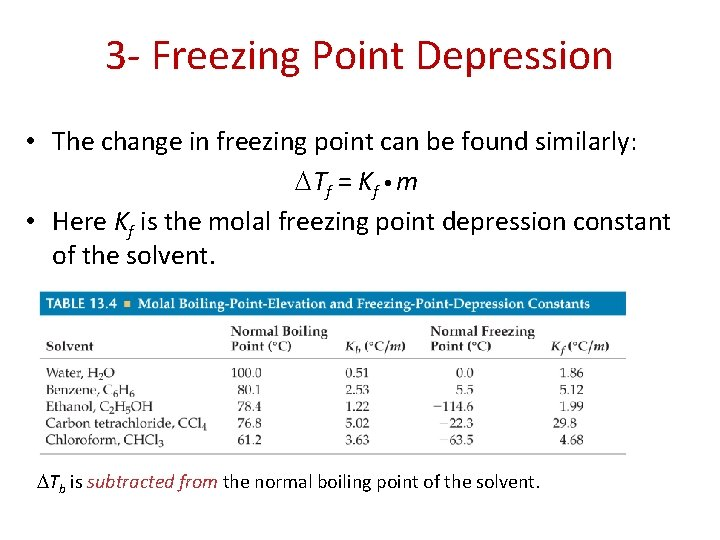3 - Freezing Point Depression • The change in freezing point can be found similarly: Tf = Kf m • Here Kf is the molal freezing point depression constant of the solvent. Tb is subtracted from the normal boiling point of the solvent.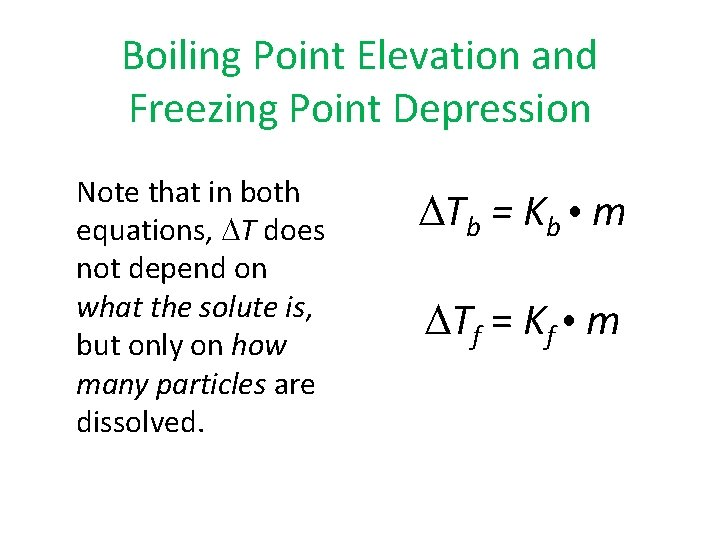Boiling Point Elevation and Freezing Point Depression Note that in both equations, T does not depend on what the solute is, but only on how many particles are dissolved. Tb = Kb m Tf = Kf m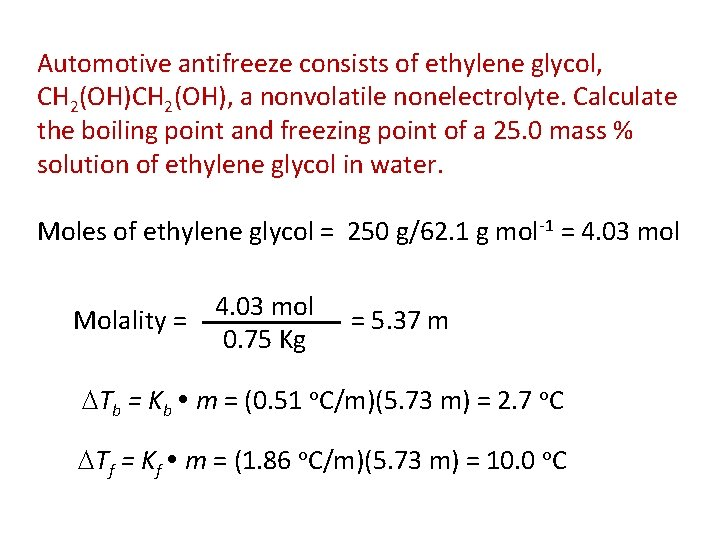Automotive antifreeze consists of ethylene glycol, CH 2(OH), a nonvolatile nonelectrolyte. Calculate the boiling point and freezing point of a 25. 0 mass % solution of ethylene glycol in water. Moles of ethylene glycol = 250 g/62. 1 g mol-1 = 4. 03 mol Molality = 4. 03 mol 0. 75 Kg = 5. 37 m Tb = Kb m = (0. 51 o. C/m)(5. 73 m) = 2. 7 o. C Tf = Kf m = (1. 86 o. C/m)(5. 73 m) = 10. 0 o. C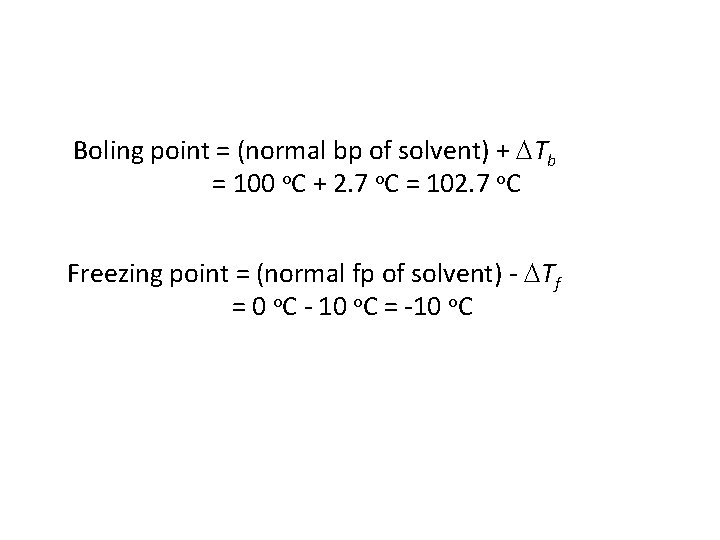Boling point = (normal bp of solvent) + Tb = 100 o. C + 2. 7 o. C = 102. 7 o. C Freezing point = (normal fp of solvent) - Tf = 0 o. C - 10 o. C = -10 o. C4 - Osmotic Pressure Osmosis • Some substances form semipermeable membranes, allowing some smaller particles to pass through, but blocking other larger particles. • In biological systems, most semipermeable membranes allow water to pass through, but solutes are not free to do so.Osmosis In osmosis, there is net movement of solvent from the area of higher solvent concentration (lower solute concentration) to the are of lower solvent concentration (higher solute concentration).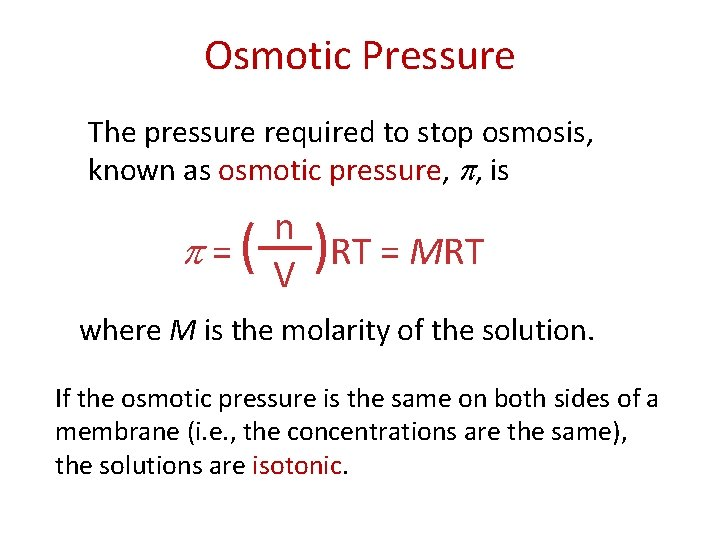Osmotic Pressure The pressure required to stop osmosis, known as osmotic pressure, , is =( n ) RT = MRT V where M is the molarity of the solution. If the osmotic pressure is the same on both sides of a membrane (i. e. , the concentrations are the same), the solutions are isotonic.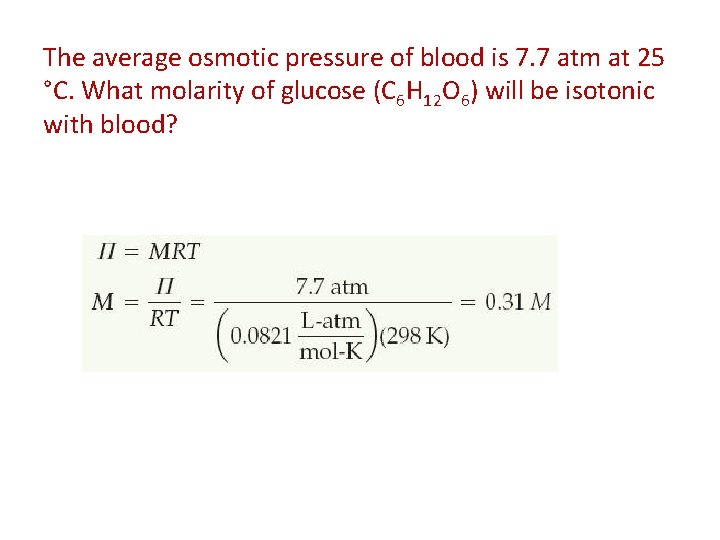The average osmotic pressure of blood is 7. 7 atm at 25 °C. What molarity of glucose (C 6 H 12 O 6) will be isotonic with blood?Using Colligative Properties to Determine Molar Mass 1. A 7. 85 g sample of a compound with the empirical formula C 5 H 4 is dissolved in 301 g of benzene. The freezing point of the solution is 1. 05° C below that of pure benzene. What are the molar mass and molecular formula of this compound? 2. A solution is prepared by dissolving 35. 0 g of hemoglobin (Hb) in enough water to make up 1 L in volume. If the osmotic pressure of the solution is found to be 10. 0 mm. Hg at 25° C, calculate the molar mass of hemoglobin.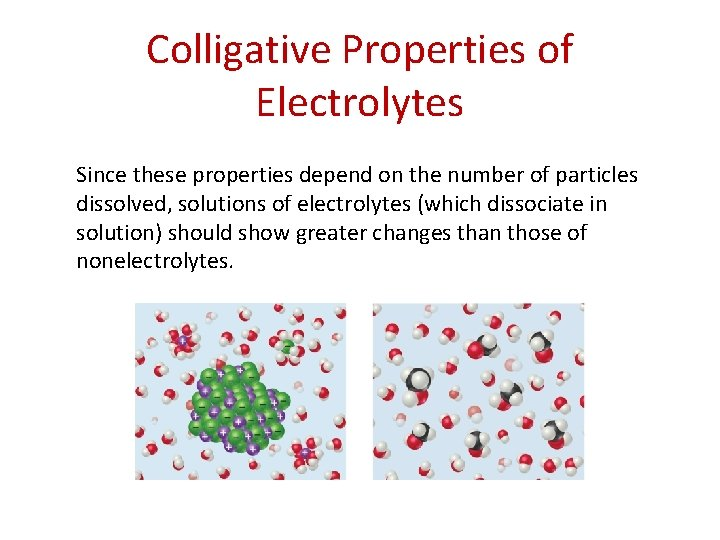Colligative Properties of Electrolytes Since these properties depend on the number of particles dissolved, solutions of electrolytes (which dissociate in solution) should show greater changes than those of nonelectrolytes.Colligative Properties of Electrolytes However, a 1 M solution of Na. Cl does not show twice the change in freezing point that a 1 M solution of methanol does.van’t Hoff Factor One mole of Na. Cl in water does not really give rise to two moles of ions.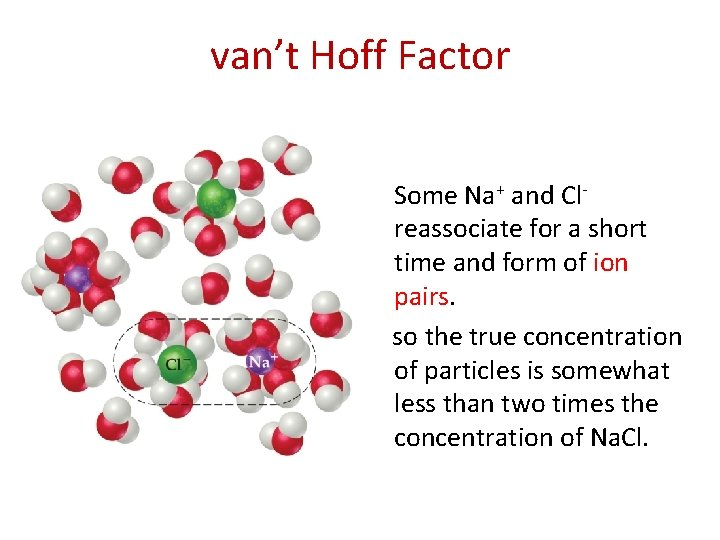van’t Hoff Factor Some Na+ and Clreassociate for a short time and form of ion pairs. so the true concentration of particles is somewhat less than two times the concentration of Na. Cl.van’t Hoff Factor • Reassociation is more likely at higher concentration. • Therefore, the number of particles present is concentrationdependent.van’t Hoff Factor The vant’t Hoff Factor of 0. 100 m for Electrolyte Solutions at 25° C Electrolyte i (Measured) i (Calculated) Sucrose 1. 0 1 Na. Cl 1. 87 2 K 2 SO 4 2. 32 3 Mg. SO 4 1. 21 2 • We modify the previous equations by multiplying by the van’t Hoff factor, i. Tf = Kf m i = i. MRT Tf = Kf m i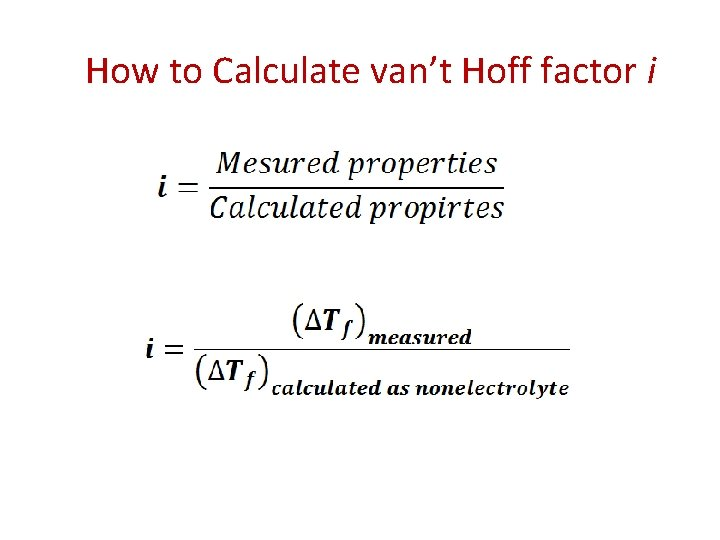How to Calculate van’t Hoff factor iThe osmotic pressure of a 0. 010 M potassium iodide (KI) solution at 25 °C is 0. 465 atm. Calculate the van’t Hoff factor for KI at this concentration. Measured Osmotic pressure (π) is 0. 465 atm Calculated Osmotic pressure (π) : π = MRT = 0. 010 X 0. 0821 X 298 = 0. 245 atm